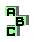SYMMEDIAN LINES

 d second Brocard triangle r9 circumcenter, second Brocard triangle r22 first Brocard triangle, medians d Brocard ellipse d second Brocard parabola d symmedian point r186 cosymmedian triangle, symmedian point, perspective r223 Johnson triangle, circumcircle r261 orthic triangle r391 r529 circumcenter, Kosnita point r557 medians, Simson line, circumcircle r645 symmedian point, centroid, Fermat points r799 medial triangle, circumcenter, orthic triangle r845 circumcircle, medians, orthic axis r960 centroid, symmedian point, Fermat points, orthocentroidal circle, Apollonian circles r961 orthocenter, symmedian point, Fermat points, orthocentroidal circle, Apollonian circles c36 Euler line r1160 cevian triangle r1332 medians, Brocard points r1439 circumcircle, Apollonian circles, symmedian point, radical center